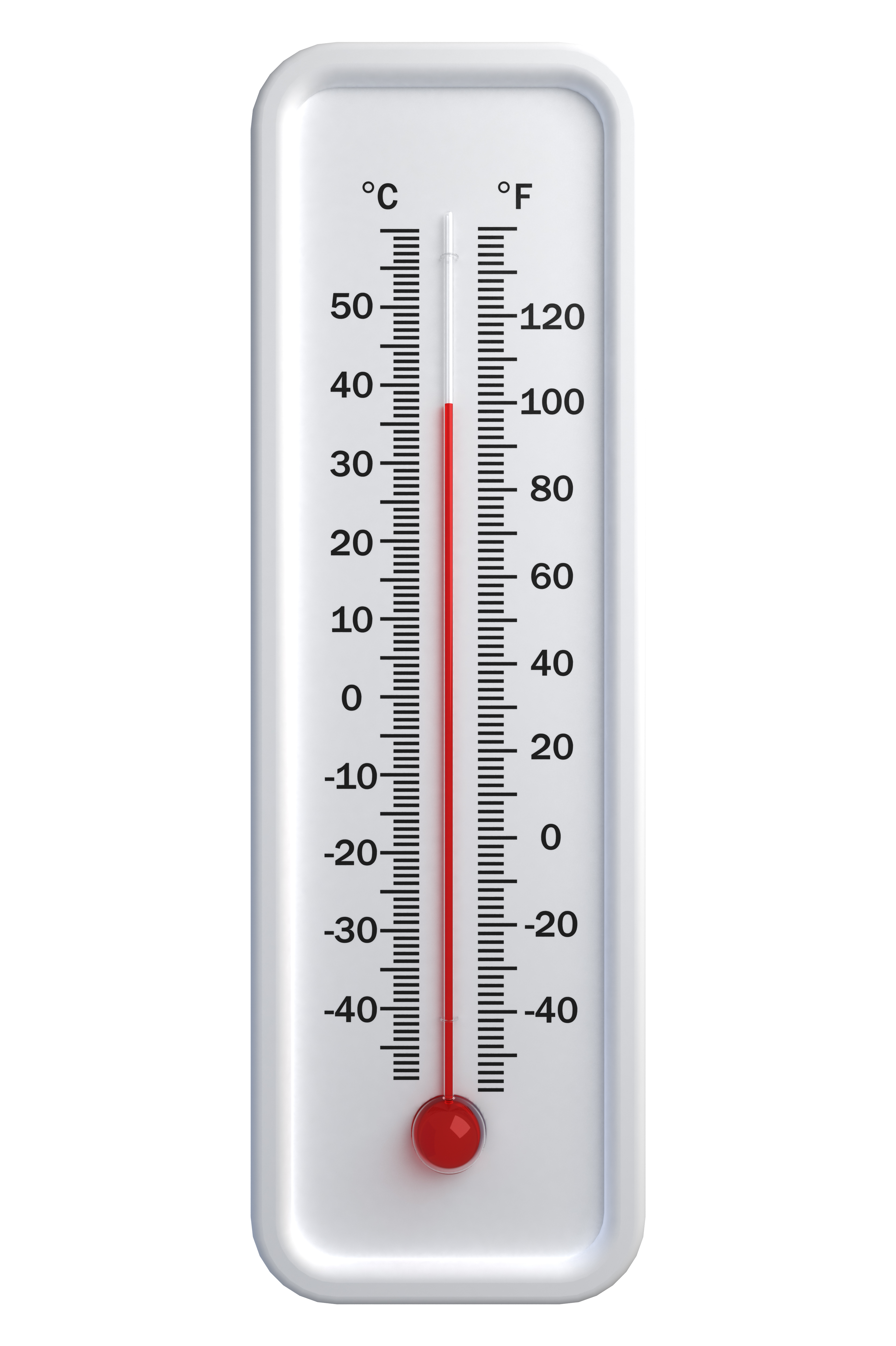In some countries temperature is measured in degrees Celsius (originally called degrees Centigrade) and in other countries it is measured in degrees Fahrenheit.The freezing point of water is 0 degrees Celsius and 32 degrees Fahrenheit.
The boiling point of water is 100 degrees Celsius and 212 degrees Fahrenheit.

Draw a graph to convert Celsius to Fahrenheit by using the above information to plot two points and joining them by a straight line.

What is the equation of this line?

Use this equation to write down an expression for converting Fahrenheit readings into Celsius.
From the same equation write down an expression for converting Celsius readings into Fahrenheit.

A temperature for the human body of between 97 and 99 degrees Fahrenheit is considered normal and a temperature of over 100 degrees Fahrenheit is said to be a fever. Find the equivalent temperatures in degrees Celsius.

What equation would you solve to find the temperature at which  Celsius and Fahrenheit readings are the same?

Click here for The Notes for Teachers and here for an NRICH poster.